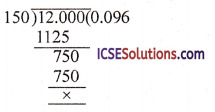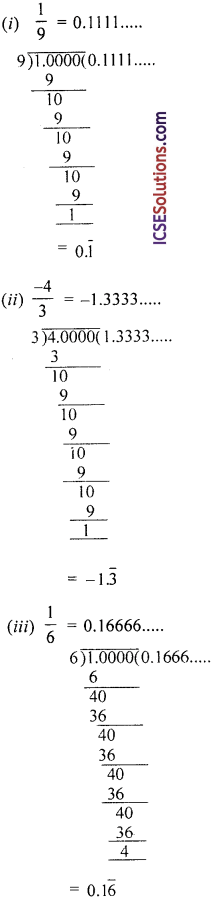Practicing OP Malhotra Class 9 Solutions Chapter 1 Rational and Irrational Numbers Ex 1(A) is the ultimate need for students who intend to score good marks in examinations.

## S Chand Class 9 ICSE Maths Solutions Chapter 1 Rational and Irrational Numbers Ex 1(A)

Question 1.
(i) Find a rational number between $$\frac { 1 }{ 2 }$$ and $$\frac { 3 }{ 4 }$$.
(ii) Find two rational numbers between 0.1 and 0.2.
(iii) How many rational numbers can you find between two given rational numbers?
Solution:
(i) One rational number between $$\frac { 1 }{ 2 }$$ and $$\frac { 3 }{ 4 }$$
= $$\frac{1}{2}\left[\frac{1}{2}+\frac{3}{4}\right]=\frac{1}{2}\left[\frac{2+3}{4}\right]$$
= $$\frac{1}{2} \times \frac{5}{4}=\frac{5}{8}$$

(ii) Two rational numbers between 0.1 and 0.2 1
First number = $$\frac { 1 }{ 2 }$$ [0.1 + 0.2]
= $$\frac { 1 }{ 2 }$$ x 0.3 = 0.15 or $$\frac { 15 }{ 100 }$$ = $$\frac { 3 }{ 20 }$$
and second number = $$\frac{1}{2}\left[\frac{3}{20}+\frac{2}{10}\right]$$
= $$\frac{1}{2}\left[\frac{3+4}{20}\right]=\frac{1}{2} \times \frac{7}{20}=\frac{7}{40}$$
∴ Two numbers are $$\frac { 3 }{ 10 }$$ and $$\frac { 7 }{ 40 }$$

(iii) We can find infinite numbers of rational numbers between two given rational numbers.

Question 2.
Find two rational numbers between
(i) $$\frac { 4 }{ 5 }$$ and $$\frac { 7 }{ 13 }$$
(ii) $$\frac { 3 }{ 4 }$$ and 1$$\frac { 1 }{ 5 }$$
Solution:
(i) One rational number between $$\frac { 4 }{ 5 }$$ and $$\frac { 7 }{ 13 }$$
= $$\frac{1}{2}\left[\frac{4}{5}+\frac{7}{13}\right]$$ $$\frac { 1 }{ 2 }$${a + b}
= $$\frac{1}{2}\left(\frac{52+35}{65}\right)=\frac{87}{130}$$
and second rational number
= $$\frac{1}{2}\left[\frac{87}{130}+\frac{7}{13}\right]=\frac{1}{2}\left[\frac{87+70}{130}\right]$$
= $$\frac{1}{2}\left[\frac{157}{130}\right]=\frac{157}{260}$$

(ii) One rational number between $$\frac { 3 }{ 4 }$$ and 1$$\frac { 1 }{ 5 }$$ or $$\frac { 3 }{ 4 }$$ and $$\frac { 6 }{ 5 }$$
= $$\frac{1}{2}\left[\frac{3}{4}+\frac{6}{5}\right]=\frac{1}{2}\left[\frac{15+24}{20}\right]$$
= $$\frac{1}{2}\left[\frac{39}{20}\right]=\frac{39}{40}$$
and second rational number
= $$\frac{1}{2}\left[\frac{39}{40}+\frac{6}{5}\right]$$
= $$\frac{1}{2}\left[\frac{39+48}{40}\right]=\frac{1}{2} \times \frac{87}{40}=\frac{87}{80}$$Question 3.
Find three rational numbers between 0 and 0.2.
Solution:
First rational number between 0 and 0.2 = $$\frac { 1 }{ 2 }$$ [0 + 0.2] = 0.1
Second rational number = $$\frac { 1 }{ 2 }$$ [0 + 0.1]
= $$\frac { 1 }{ 2 }$$ [0.1] = 0.05
and third rational number
= $$\frac { 1 }{ 2 }$$ [0.1 + 0.2] = $$\frac { 1 }{ 2 }$$ [0.3]
= 0.15
Hence three rational numbers are 0.05, 0.1 and 0.15

Question 4.
Find three rational numbers between 3 and 4.
Solution:
First rational number between 3 and 4
= $$\frac { 1 }{ 2 }$$ [3 + 4] = $$\frac { 1 }{ 2 }$$ x 7 = $$\frac { 7 }{ 2 }$$
Second rational number between 3 and $$\frac { 7 }{ 2 }$$
= $$\frac{1}{2}\left[3+\frac{7}{2}\right]=\frac{1}{2} \times \frac{6+7}{2}=\frac{13}{4}$$
and third number between $$\frac { 7 }{ 2 }$$ and 4
= $$\frac{1}{2}\left[\frac{7}{2}+4\right]=\frac{1}{2}\left[\frac{7+8}{2}\right]=\frac{1}{2} \times \frac{15}{2}=\frac{15}{4}$$
Hence three rational number are
$$\frac { 13 }{ 4 }$$, $$\frac { 7 }{ 2 }$$ and $$\frac { 15 }{ 4 }$$

Question 5.
Find the rational number that is one seventh of the way from 1$$\frac { 3 }{ 4 }$$ to 4$$\frac { 3 }{ 8 }$$.
Solution:
1$$\frac{3}{4} \text { to } 4 \frac{3}{8} \Rightarrow \frac{7}{4} \text { to } \frac{35}{8}$$
⇒ $$\frac { 14 }{ 8 }$$ to $$\frac { 35 }{ 8 }$$
Between 14 and 35, there are 21 terms i.e.
$$\frac{15}{8}, \frac{16}{8}, \frac{17}{8}, \frac{18}{8} \ldots . ., \frac{34}{8}$$
∴ $$\frac { 1 }{ 7 }$$th of 21 terms = 21 x $$\frac { 1 }{ 7 }$$ = 3rd
∴ 7th term = $$\frac { 17 }{ 8 }$$ i.e. 2$$\frac { 1 }{ 8 }$$.

Question 6.
Find four rational numbers between – 1 and $$\frac { 1 }{ -2 }$$.
Solution:
First rational number between – 1 and $$\frac { 1 }{ -2 }$$Question 7.
Express $$\frac { 12 }{ 125 }$$ as decimal fraction.
Solution:
$$\frac { 12 }{ 125 }$$ = 0.096
(Dividing 12 by 125 by long division)Question 8.
Find a vulgar fraction equivalent to 0.0
Solution:
0.03 = 0.033333
Let x = 0.033333 ….
10x = 3.3333 …. (i)
100x = 3.3333 …. (ii)
Subtracting (i) from (ii)
99x = 3.00 ….
x = $$\frac { 3 }{ 90 }$$ = $$\frac { 1 }{ 30 }$$
∴ Required vulgar fraction = $$\frac { 1 }{ 10 }$$Question 9.
Express the following rational numbers in the form $$\frac { p }{ q }$$, p, q are integers, q ≠ 0.
(i) 6.$$\overline{46}$$
(ii) 0.1$$\overline{36}$$
(iii) 3.$$\overline{146}$$
(iv) – 5.$$\overline{12}$$
Solution:
(i) 6.$$\overline{46}$$ = 6.464646 ….
Let x = 6.46464646 …. (i)
100x = 646.46464646 ….(ii)
Subtracting (i) from (ii)
99x = 646 – 6 = 640
x = $$\frac { 640 }{ 99 }$$
∴ Fraction = $$\frac { 640 }{ 99 }$$

(ii) 0.1$$\overline{36}$$ = 0.1363636…
Let x = 0.1363636….
10x = 1.363636… (i)
and 1000c = 136.363636 ….(ii)
Subtracting (i) from (ii)
990x = 135
x = $$\frac{135}{990}=\frac{27}{198}=\frac{3}{22}$$
∴ Fraction = $$\frac { 3 }{ 22 }$$

(iii) 3.$$\overline{146}$$
Let x = 3.$$\overline{146}$$ = 3.146146146… (i)
1000x = 3146.146146146…. (ii)
Subtracting (i) from (ii)
999x = 3143
x = $$\frac { 3143 }{ 999 }$$
Hence fraction = $$\frac { 3143 }{ 999 }$$

(iv) – 5.$$\overline{12}$$
Let x = – 5.$$\overline{12}$$ = – 5.121212 … (i)
100x = – 512.121212 ….(ii)
Subtracting (i) from (ii)
99x = 507
⇒ x = $$\frac{-507}{99}=\frac{-169}{33}$$
∴ Fraction = $$\frac { -169 }{ 33 }$$

Question 10.
Write the terminating decimal numeral for the given rational number :
(i) $$\frac { 7 }{ 4 }$$
(ii) $$\frac { 29 }{ 50 }$$
(iii) $$\frac { 17 }{ 32 }$$
Solution:Question 11.
Write the repeating decimal for each of the following and use a bar to show the repetend.
(i) $$\frac { 1 }{ 9 }$$
(ii) $$\frac { -4 }{ 3 }$$
(iii) $$\frac { 1 }{ 6 }$$
Solution: